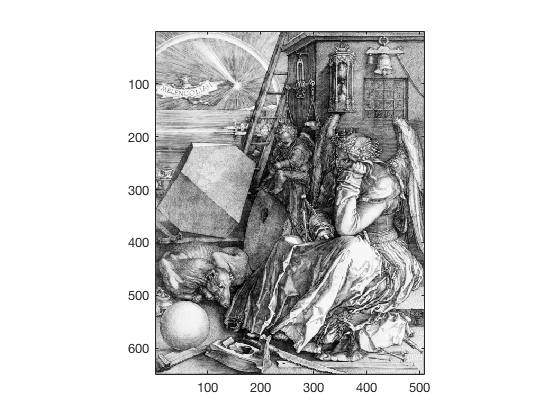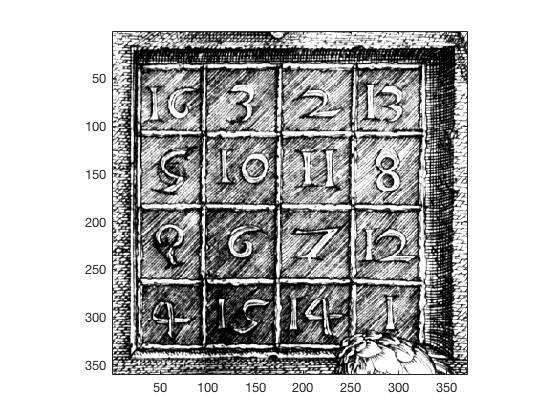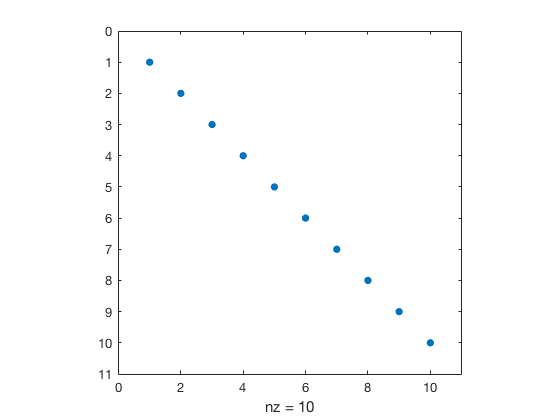# Lecture 6: Matrix¶

Date: 09/19/2017, Tuessday

In :

format compact


## Matrix operation basics¶

Making a magic square

In :

A = magic(3)

A =
8     1     6
3     5     7
4     9     2


Take transpose

In :

A'

ans =
8     3     4
1     5     9
6     7     2


Rotate by 90 degree. (Not so useful for linear algebra. Could be useful for image processing.)

In :

rot90(A')

ans =
4     9     2
3     5     7
8     1     6


Sum over each column

In :

sum(A)

ans =
15    15    15


Another equivalent way

In :

sum(A, 1)

ans =
15    15    15


Sum over each row

In :

sum(A, 2)

ans =
15
15
15


Extract the diagonal elements.

In :

diag(A)

ans =
8
5
2


The sum of diagonal elements is also 15, by the definition of a magic square.

In :

sum(diag(A))

ans =
15


Determinant

In :

det(A)

ans =
-360


Matrix inversion $$A^{-1}$$

In :

inv(A)

ans =
0.1472   -0.1444    0.0639
-0.0611    0.0222    0.1056
-0.0194    0.1889   -0.1028


## Built-in image for magic square¶

In :

load durer
image(X)
colormap(map)
axis imageIn :

load detail
image(X)
colormap(map)
axis image## Vector norms¶

In :

x = 1:5 % make a boring vector

x =
1     2     3     4     5


Calculate p-norm from formula

In :

my_norm = @(x,p) (sum(abs(x).^p))^(1/p)

my_norm =
function_handle with value:
@(x,p)(sum(abs(x).^p))^(1/p)


Check if it works.

In :

my_norm(x,1)

ans =
15


As p increases, the norm converges to $$\max(|x|)$$

In :

for p=1:10
my_norm(x,p)
end

ans =
15
ans =
7.4162
ans =
6.0822
ans =
5.5937
ans =
5.3602
ans =
5.2321
ans =
5.1557
ans =
5.1073
ans =
5.0756
ans =
5.0541


It blows up at $$p=442$$ because $$5^{442}$$ exceeds the realmin.

In :

my_norm(x,441), my_norm(x,442)

ans =
5
ans =
Inf

In :

5^441

ans =
1.7611e+308

In :

5^442

ans =
Inf


Built-in function norm works for large numbers, though. Think about why.

In :

norm(x,441), norm(x,442)

ans =
5
ans =
5


## Conditioning¶

4x4 magic square

In :

B = magic(4)

B =
16     2     3    13
5    11    10     8
9     7     6    12
4    14    15     1


It is singular and ill-conditioned.

In :

inv(B)

Warning: Matrix is close to singular or badly scaled. Results may be inaccurate. RCOND =  4.625929e-18.
ans =
1.0e+15 *
-0.2649   -0.7948    0.7948    0.2649
-0.7948   -2.3843    2.3843    0.7948
0.7948    2.3843   -2.3843   -0.7948
0.2649    0.7948   -0.7948   -0.2649

In :

det(B)

ans =
5.1337e-13

In :

cond(B)

ans =
4.7133e+17


Use 1-norm instead. All norms should have similar order of magnitude.

In :

cond(B,1)

Warning: Matrix is close to singular or badly scaled. Results may be inaccurate. RCOND =  4.625929e-18.
> In cond (line 46)
ans =
2.1617e+17


The condition number reaches 1/eps, leading to large numerical error.

In :

1/eps

ans =
4.5036e+15


## Sparse matrix¶

In :

A = eye(10)

A =
1     0     0     0     0     0     0     0     0     0
0     1     0     0     0     0     0     0     0     0
0     0     1     0     0     0     0     0     0     0
0     0     0     1     0     0     0     0     0     0
0     0     0     0     1     0     0     0     0     0
0     0     0     0     0     1     0     0     0     0
0     0     0     0     0     0     1     0     0     0
0     0     0     0     0     0     0     1     0     0
0     0     0     0     0     0     0     0     1     0
0     0     0     0     0     0     0     0     0     1


Store it in the sparse form saves memory.

In :

As = sparse(A)

As =
(1,1)        1
(2,2)        1
(3,3)        1
(4,4)        1
(5,5)        1
(6,6)        1
(7,7)        1
(8,8)        1
(9,9)        1
(10,10)       1


Use whos A and whos As to check memory usage.

Visualize sparsity. Also works for As

In :

spy(A)